# Python股市数据分析教程——学会它，或可以实现半“智能”炒股 (Part 2)

+关注继续查看

## 交易策略

• 当短期均线越过长期均线时，交易金融资产。
• 当短期均线再一次越过长期均线时，结束交易。

apple['20d-50d'] = apple['20d'] - apple['50d']
apple.tail()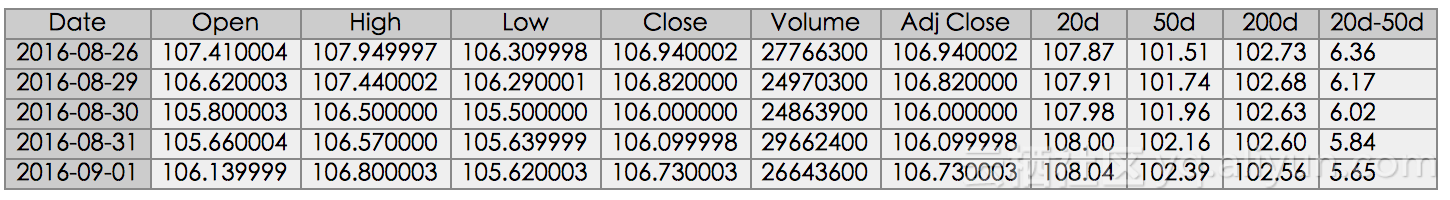apple["Regime"] = np.where(apple['20d-50d'] > 0, 1, 0)
apple["Regime"] = np.where(apple['20d-50d'] < 0, -1, apple["Regime"])
apple.loc['2016-01-01':'2016-08-07',"Regime"].plot(ylim = (-2,2)).axhline(y = 0, color = "black", lw = 2)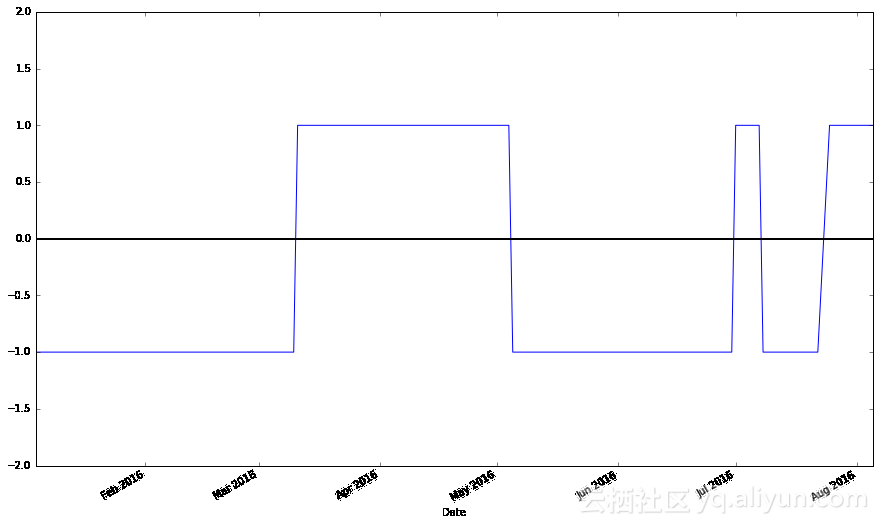apple["Regime"].plot(ylim = (-2,2)).axhline(y = 0, color = "black", lw = 2)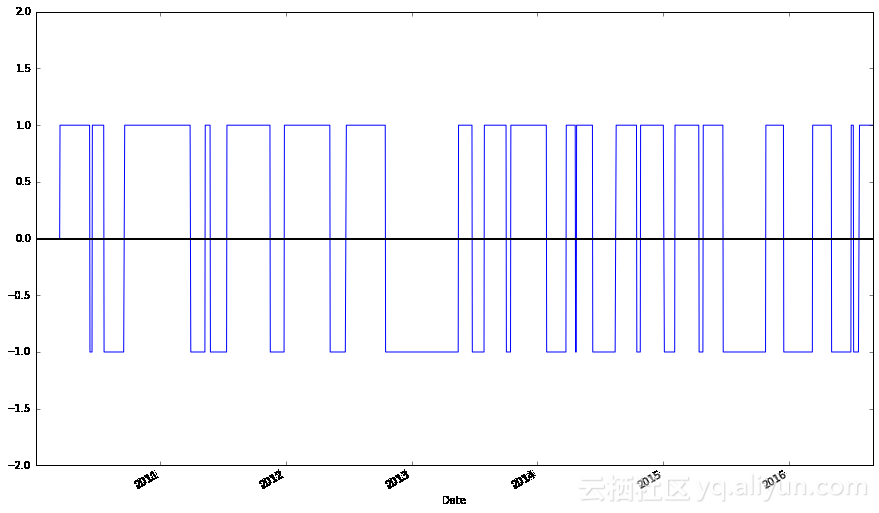apple["Regime"].value_counts()
1       966
-1      663
0       50
Name: Regime, dtype: int64

st = sing(rt - rt-1)

st ∈ {-1, 0, 1}，其中-1表示"抛出"，1表示"买入"，0表示不采取任何措施，我们可以这样获取信号：

regime_orig = apple.ix[-1, "Regime"]
apple.ix[-1, "Regime"] = 0
apple["Signal"] = np.sign(apple["Regime"] - apple["Regime"].shift(1))
apple.ix[-1, "Regime"] = regime_orig
apple.tail()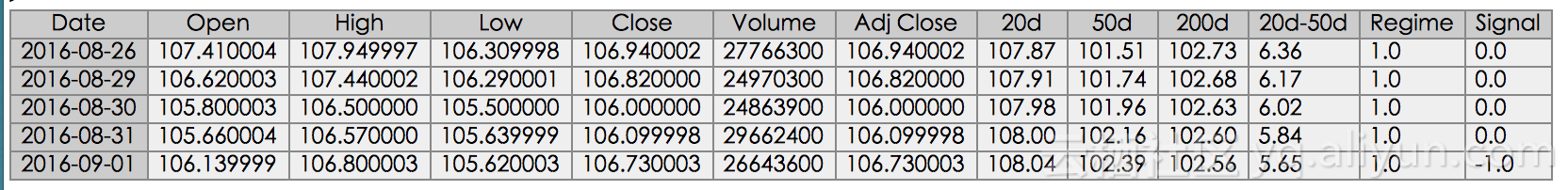apple["Signal"].plot(ylim = (-2, 2))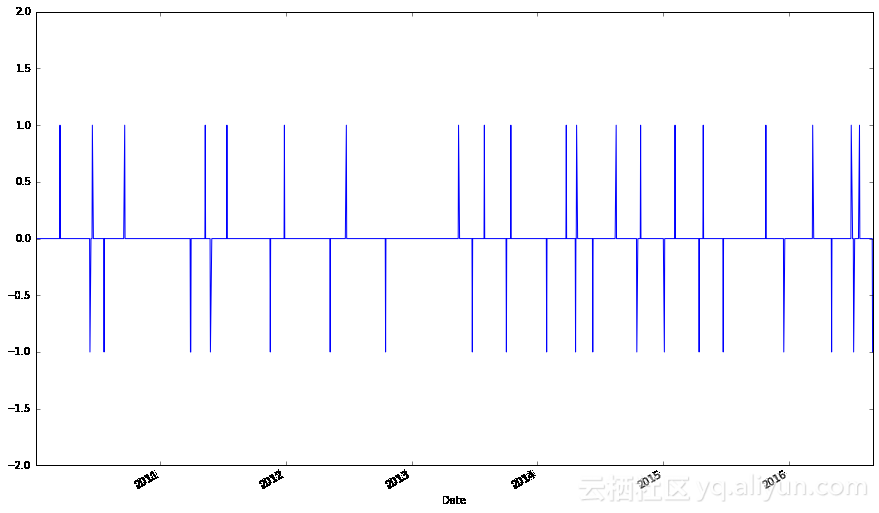apple["Signal"].value_counts()
0.0     1637
-1.0    21
1.0     20
Name: Signal, dtype: int64

apple.loc[apple["Signal"] == 1, "Close"]
Date
2010-03-16    224.449997
2010-06-18    274.070011
2010-09-20    283.230007
2011-05-12    346.569988
2011-07-14    357.770004
2011-12-28    402.640003
2012-06-25    570.770020
2013-05-17    433.260010
2013-07-31    452.529984
2013-10-16    501.110001
2014-03-26    539.779991
2014-04-25    571.939980
2014-08-18     99.160004
2014-10-28    106.739998
2015-02-05    119.940002
2015-04-28    130.559998
2015-10-27    114.550003
2016-03-11    102.260002
2016-07-01     95.889999
2016-07-25     97.339996
Name: Close, dtype: float64
apple.loc[apple["Signal"] == -1, "Close"]
Date
2010-06-11    253.509995
2010-07-22    259.020000
2011-03-30    348.630009
2011-03-31    348.510006
2011-05-27    337.409992
2011-11-17    377.410000
2012-05-09    569.180023
2012-10-17    644.610001
2013-06-26    398.069992
2013-10-03    483.409996
2014-01-28    506.499977
2014-04-22    531.700020
2014-06-11     93.860001
2014-10-17     97.669998
2015-01-05    106.250000
2015-04-16    126.169998
2015-06-25    127.500000
2015-12-18    106.029999
2016-05-05     93.239998
2016-07-08     96.680000
2016-09-01    106.730003
Name: Close, dtype: float64
apple_signals = pd.concat([
pd.DataFrame({"Price": apple.loc[apple["Signal"] == 1, "Close"],
"Regime": apple.loc[apple["Signal"] == 1, "Regime"],
pd.DataFrame({"Price": apple.loc[apple["Signal"] == -1, "Close"],
"Regime": apple.loc[apple["Signal"] == -1, "Regime"],
"Signal": "Sell"}),
])
apple_signals.sort_index(inplace = True)
apple_signals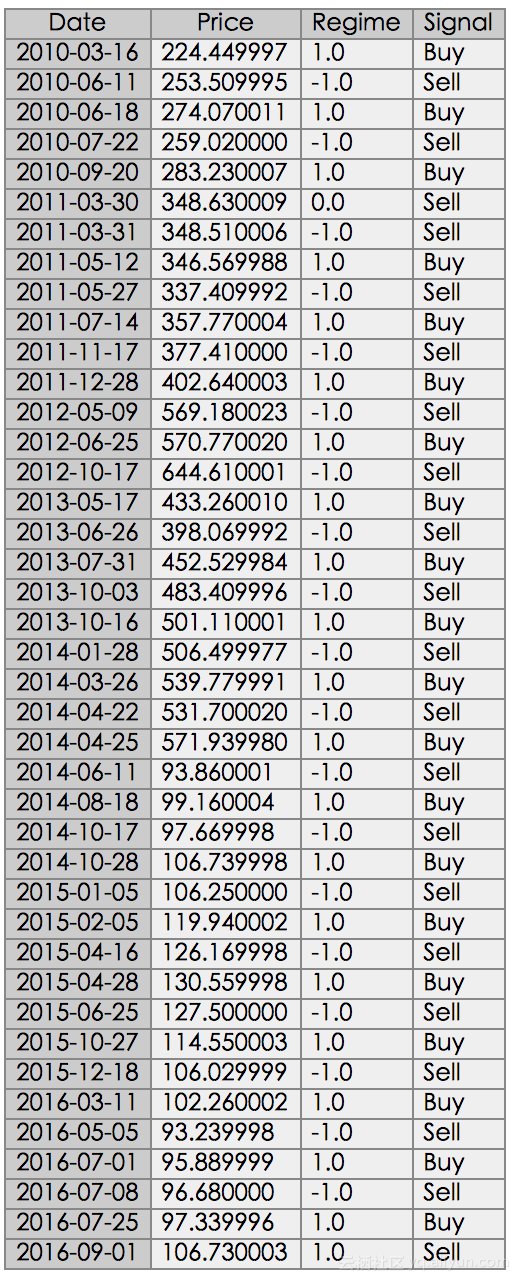apple_long_profits = pd.DataFrame({
apple_signals["Regime"] == 1, "Price"],
"Profit": pd.Series(apple_signals["Price"] - apple_signals["Price"].shift(1)).loc[
apple_signals.loc[(apple_signals["Signal"].shift(1) == "Buy") & (apple_signals["Regime"].shift(1) == 1)].index
].tolist(),
"End Date": apple_signals["Price"].loc[
apple_signals.loc[(apple_signals["Signal"].shift(1) == "Buy") & (apple_signals["Regime"].shift(1) == 1)].index
].index
})
apple_long_profits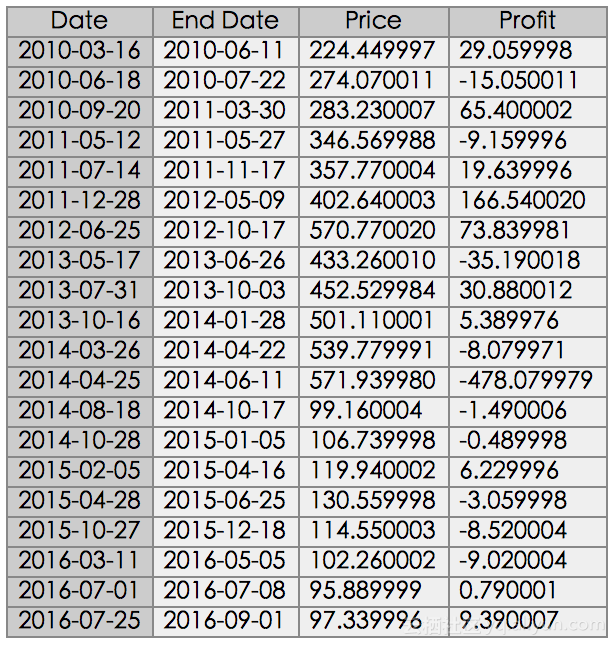pandas_candlestick_ohlc(apple, stick = 45, otherseries = ["20d", "50d", "200d"])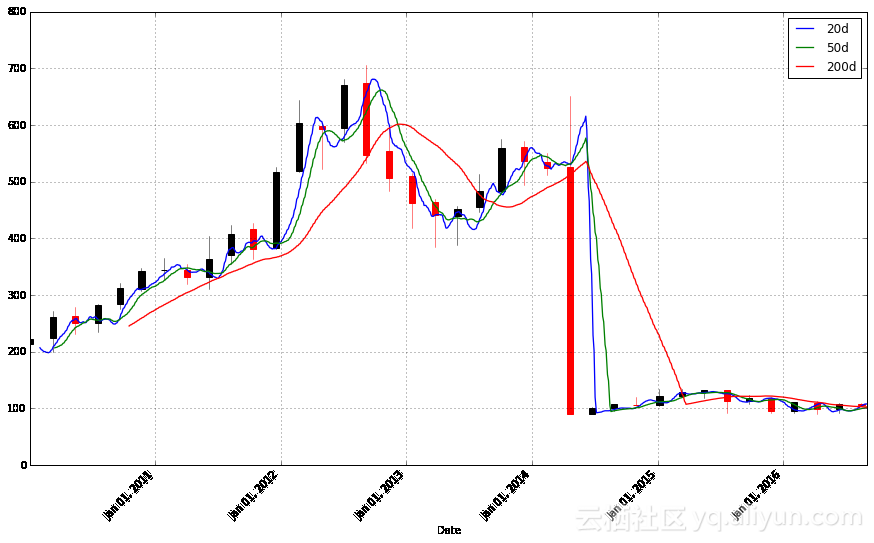def ohlc_adj(dat):
return pd.DataFrame({"Open": dat["Open"] * dat["Adj Close"] / dat["Close"],
"High": dat["High"] * dat["Adj Close"] / dat["Close"],
"Low": dat["Low"] * dat["Adj Close"] / dat["Close"],

"Signal": "Sell"}),
])
].tolist(),
].index
})

pandas_candlestick_ohlc(apple_adj, stick = 45, otherseries = ["20d", "50d", "200d"])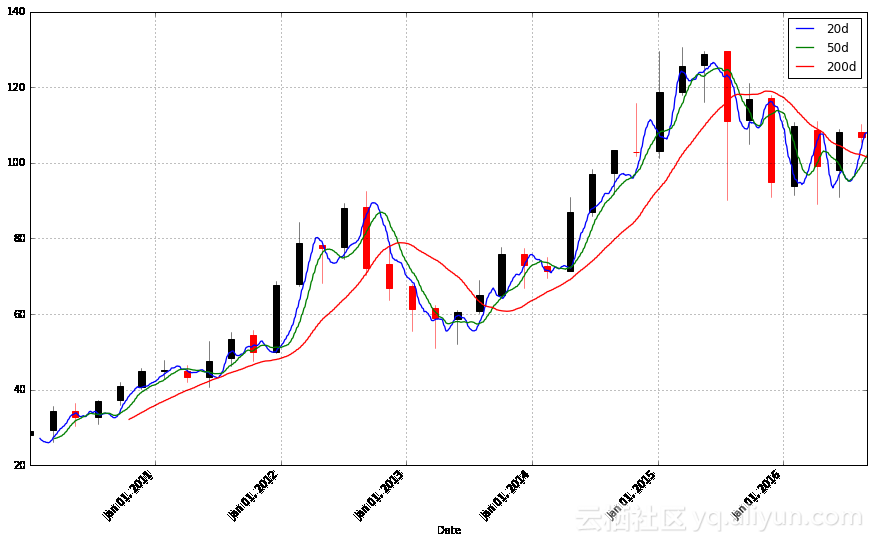apple_adj_long_profits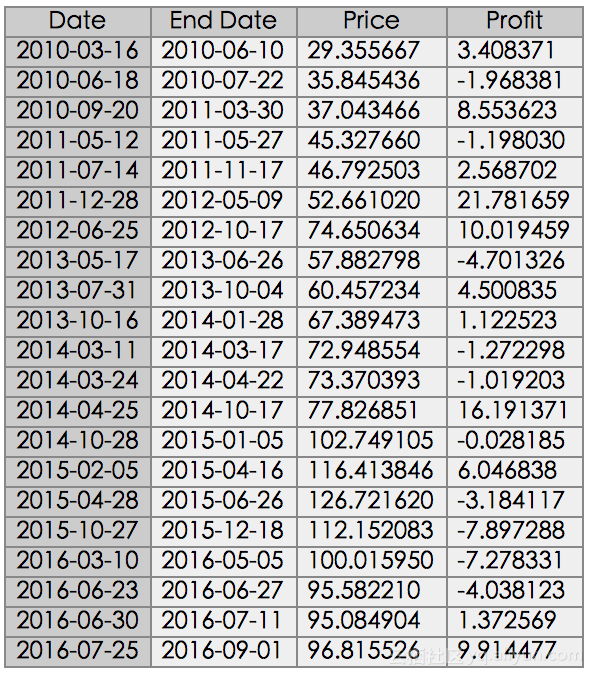• 在任何交易中，仅投资所有投资总额的10%。
• 如果损失超过交易金额的20%，则退出仓位。

• 股票交易以100股为单位。
• 我们的止损规则包含在股价下跌至一定程度时将股票抛出的指令。因此，我们需要检查这一期间的低价是否已经足够得低，以至于触发止损指令。实际上，除非我们买入了看跌期权，否则我们无法保证以设置的止损价格抛出股票，但为简单起见，我们将这个价格作为抛出价。
• 每一笔交易都需要向经纪人支付一笔佣金，这部分费用应该计算在内。但在这里我们不这样做。

tradeperiods = pd.DataFrame({"Start": apple_adj_long_profits.index,
apple_adj_long_profits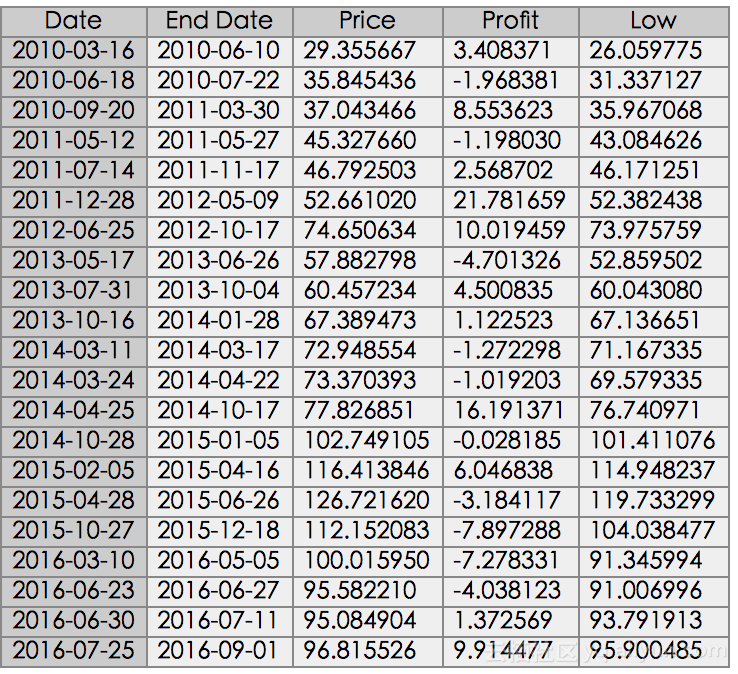cash = 1000000
apple_backtest = pd.DataFrame({"Start Port. Value": [],
"End Port. Value": [],
"End Date": [],
"Shares": [],
"Share Price": [],
"Profit per Share": [],
"Total Profit": [],
"Stop-Loss Triggered": []})
port_value = .1
batch = 100
stoploss = .2
batches = np.floor(cash * port_value) // np.ceil(batch * row["Price"]) # Maximum number of batches of stocks invested in
trade_val = batches * batch * row["Price"]
if row["Low"] < (1 - stoploss) * row["Price"]:   # Account for the stop-loss
share_profit = np.round((1 - stoploss) * row["Price"], 2)
stop_trig = True
else:
share_profit = row["Profit"]
stop_trig = False
profit = share_profit * batches * batch

apple_backtest = apple_backtest.append(pd.DataFrame({
"Start Port. Value": cash,
"End Port. Value": cash + profit,
"End Date": row["End Date"],
"Shares": batch * batches,
"Share Price": row["Price"],
"Profit per Share": share_profit,
"Total Profit": profit,
"Stop-Loss Triggered": stop_trig
}, index = [index]))
cash = max(0, cash + profit)

apple_backtest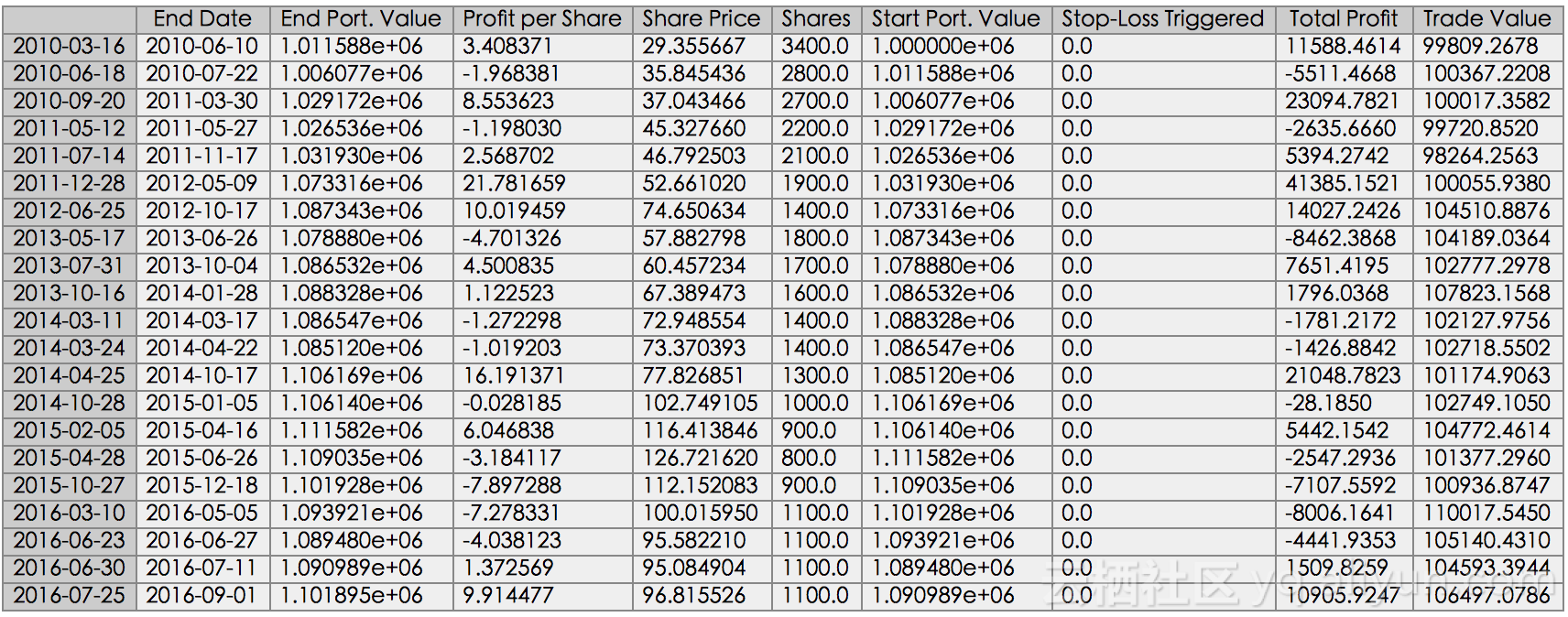apple_backtest["End Port. Value"].plot()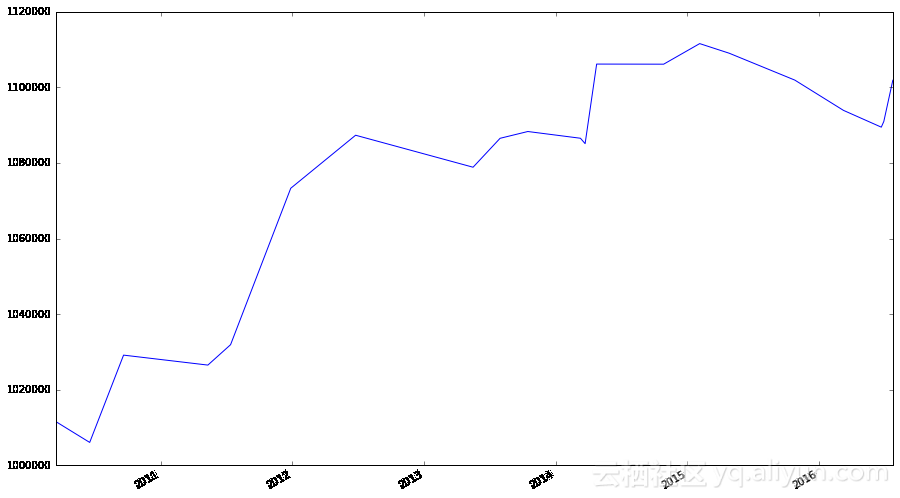def ma_crossover_orders(stocks, fast, slow):
fast_str = str(fast) + 'd'
slow_str = str(slow) + 'd'
ma_diff_str = fast_str + '-' + slow_str

trades = pd.DataFrame({"Price": [], "Regime": [], "Signal": []})
for s in stocks:
s[fast_str] = np.round(s["Close"].rolling(window = fast, center = False).mean(), 2)
s[slow_str] = np.round(s["Close"].rolling(window = slow, center = False).mean(), 2)
s[ma_diff_str] = s[fast_str] - s[slow_str]

s["Regime"] = np.where(s[ma_diff_str] > 0, 1, 0)

s["Regime"] = np.where(s[ma_diff_str] < 0, -1, s["Regime"])

regime_orig = s.ix[-1, "Regime"]
s.ix[-1, "Regime"] = 0
s["Signal"] = np.sign(s["Regime"] - s["Regime"].shift(1))

s.ix[-1, "Regime"] = regime_orig

signals = pd.concat([
pd.DataFrame({"Price": s.loc[s["Signal"] == 1, "Close"],
"Regime": s.loc[s["Signal"] == 1, "Regime"],
pd.DataFrame({"Price": s.loc[s["Signal"] == -1, "Close"],
"Regime": s.loc[s["Signal"] == -1, "Regime"],
"Signal": "Sell"}),
])
signals.index = pd.MultiIndex.from_product([signals.index, [s]], names = ["Date", "Symbol"])

def backtest(signals, cash, port_value = .1, batch = 100):
SYMBOL = 1
portfolio = dict()
port_prices = dict()

results = pd.DataFrame({"Start Cash": [],
"End Cash": [],
"Portfolio Value": [],
"Type": [],
"Shares": [],
"Share Price": [],
"Profit per Share": [],
"Total Profit": []})

for index, row in signals.iterrows():
shares = portfolio.setdefault(index[SYMBOL], 0)
batches = 0
cash_change = row["Price"] * shares
portfolio[index[SYMBOL]] = 0

old_price = port_prices.setdefault(index[SYMBOL], row["Price"])
portfolio_val = 0
for key, val in portfolio.items():
portfolio_val += val * port_prices[key]

if row["Signal"] == "Buy" and row["Regime"] == 1:
batches = np.floor((portfolio_val + cash) * port_value) // np.ceil(batch * row["Price"])
trade_val = batches * batch * row["Price"]
portfolio[index[SYMBOL]] = batches * batch
port_prices[index[SYMBOL]] = row["Price"]
old_price = row["Price"]
elif row["Signal"] == "Sell" and row["Regime"] == -1:
pass

pprofit = row["Price"] - old_price

results = results.append(pd.DataFrame({
"Start Cash": cash,
"End Cash": cash + cash_change,
"Portfolio Value": cash + cash_change + portfolio_val + trade_val,
"Type": row["Signal"],
"Shares": batch * batches,
"Share Price": row["Price"],
"Profit per Share": pprofit,
"Total Profit": batches * batch * pprofit
}, index = [index]))
cash += cash_change

results.sort_index(inplace = True)
results.index = pd.MultiIndex.from_tuples(results.index, names = ["Date", "Symbol"])

return results

microsoft = web.DataReader("MSFT", "yahoo", start, end)
netflix = web.DataReader("NFLX", "yahoo", start, end)
amazon = web.DataReader("AMZN", "yahoo", start, end)
yahoo = web.DataReader("YHOO", "yahoo", start, end)
sony = web.DataReader("SNY", "yahoo", start, end)
nintendo = web.DataReader("NTDOY", "yahoo", start, end)
ibm = web.DataReader("IBM", "yahoo", start, end)
hp = web.DataReader("HPQ", "yahoo", start, end)
signals = ma_crossover_orders([("AAPL", ohlc_adj(apple)),
fast = 20, slow = 50)
signals
bk = backtest(signals, 1000000)
bk
bk["Portfolio Value"].groupby(level = 0).apply(lambda x: x[-1]).plot()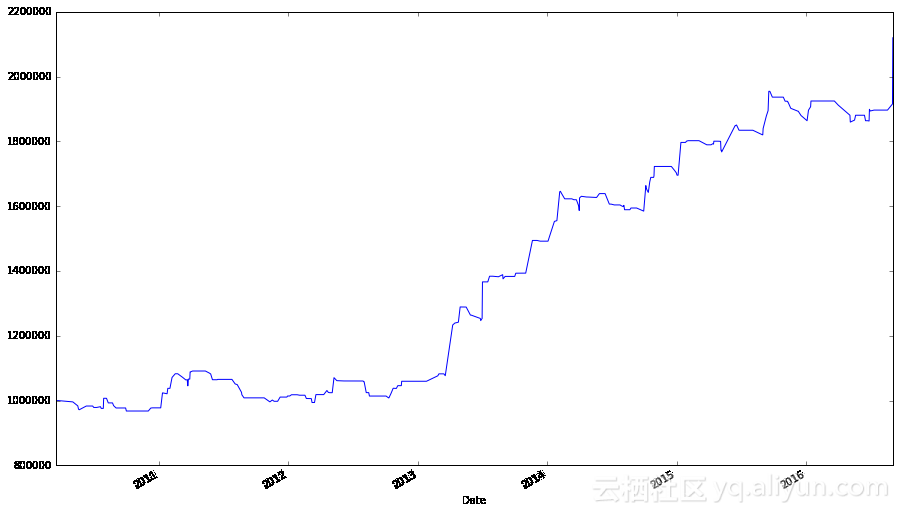## 基准测试

spyder = web.DataReader("SPY", "yahoo", start, end)
spyder.iloc[[0,-1],:]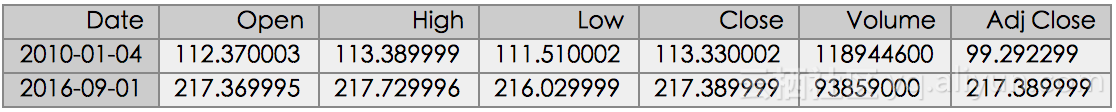batches = 1000000 // np.ceil(100 * spyder.ix[0,"Adj Close"]) # Maximum number of batches of stocks invested in
trade_val = batches * batch * spyder.ix[0,"Adj Close"] # How much money is used to buy SPY
final_val = batches * batch * spyder.ix[-1,"Adj Close"] + (1000000 - trade_val) # Final value of the portfolio
final_val
2180977.0
ax_bench = (spyder["Adj Close"] / spyder.ix[0, "Adj Close"]).plot(label = "SPY")
ax_bench = (bk["Portfolio Value"].groupby(level = 0).apply(lambda x: x[-1]) / 1000000).plot(ax = ax_bench, label = "Portfolio")
ax_bench.legend(ax_bench.get_lines(), [l.get_label() for l in ax_bench.get_lines()], loc = 'best')
ax_bench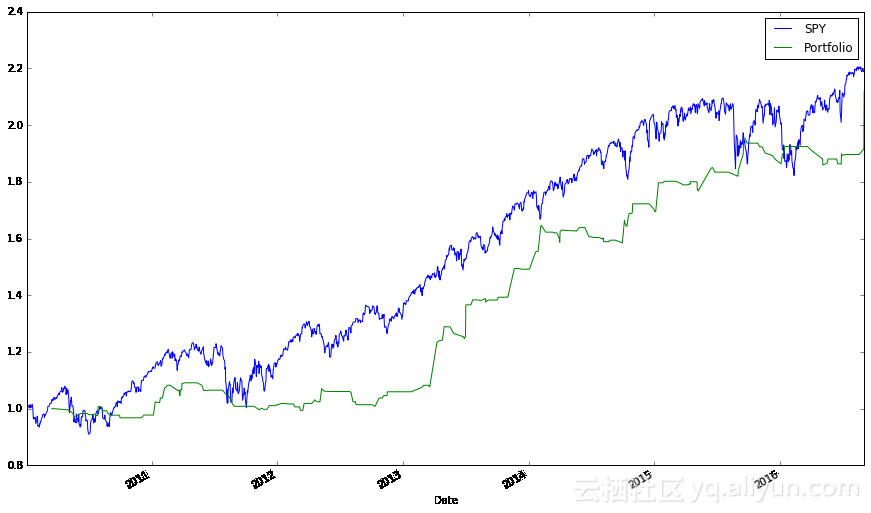• 当每月收盘价高于十月均线时，买入SPY基金。
• 当十月均线的动量为正时，买入SPY基金。（动量是移动平均过程中的第一个差值，即MOtq = MAtq - MAt-1q。）

（我最早在这里知道了这些策略。）普遍的经验仍然成立：对于一个包含大量活跃交易的复杂交易系统，如果一个涉及指数基金且不进行频繁交易的简单策略击败了它，那么不要使用这个复杂系统。实际上，这个要求很难满足。

## 问题

### 问题2

1. 当移动均线相差固定金额时，触发交易
2. 当移动均线相差一定数值的（滚动）标准差时，触发交易，标准差根据如下公式定义: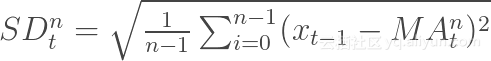（pandas确实提供了计算滚动标准差的方法。）对于后者，如果移动均线相差p x SDtn，交易信号将被释放。

### 问题31. Tushare简介1.1. 开源、免费版Tushare 　　Tushare是一个免费、开源的python财经数据接口包。主要实现对股票等金融数据从数据采集、清洗加工 到 数据存储的过程，能够为金融分析人员提供快速、整洁、和多样的便于分析的数据，为他们在数据获取方面极大地减轻工作量，使他们更加专注于策略和模型的研究与实现上。考虑到Python pandas包在金融量化分析中体现出的优势，Tushare返回的绝大部分的数据格式都是pandas DataFrame类型，非常便于用pandas/NumPy/Matplotlib进行数据分析和可视化。当然，如果您习惯了用Excel或者关系型数
0 0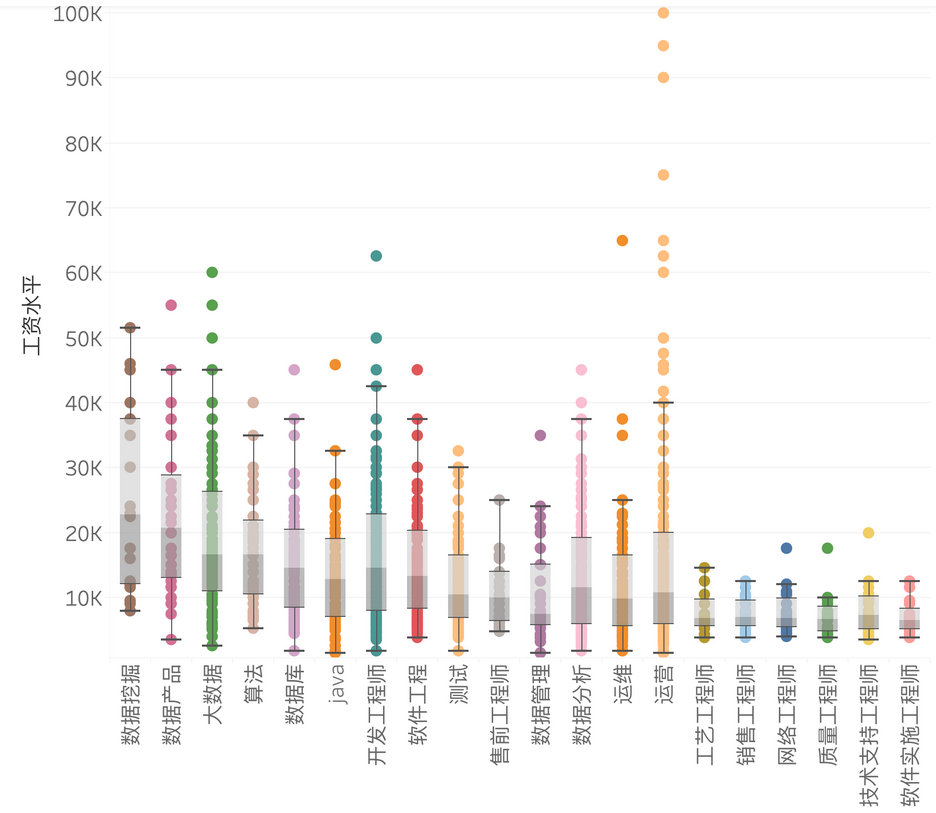「一行分析」利用12000条招聘数据分析Python学习方向和就业方向

0 0Python可视化: 历年中国大学学术排行榜
1359 02896 05000 0《数据科学实战手册（R+Python）》一第2章 汽车数据的可视化分析（R）
3051 0《Python数据分析与挖掘实战》一第1章 数据挖掘基础1.1　某知名连锁餐饮企业的困惑
1066 0Python股市数据分析教程——学会它，或可以实现半“智能”炒股 (Part 1)

26925 0
【方向】15654

【科技少年】Python绘画编程第一课252520422101

【视觉与图像】Python+OpenCV教程基础篇8614

Python数据科学手册2708

【视觉与图像】Python+OpenCV教程入门篇-1月16日更新7916

Python 系列直播——深入Python与日志服务，玩转大规模数据分析处理实战第二讲

SAS数据分析开发之道：软件质量的维度